# Fluid flow past a cylinder

Fluid flow past a cylinder is classical mathematical solution for the flow of an inviscid, incompressible fluid around a cylinder that is transverse to the flow. Far from the cylinder, the flow is unidirectional and uniform.

"The flow of an incompressible fluid past a cylinder is one of the first mathematical models that a student of fluid dynamics encounters. This flow is an excellent vehicle for the study of concepts that will be encountered numerous times in mathematical physics, such as vector fields, coordinate transformations, and most important, the physical interpretation of mathematical results." 

##  Mathematical solution

A cylinder (or disk) of radius R is placed in two-dimensional, incompressible, inviscid flow. The goal is to find the steady velocity vector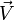$\vec{V}$ and pressure p in a plane, subject to the condition that far from the cylinder the velocity vector is$\vec{V}=U\widehat{i}+0\widehat{j}$

where U is a constant, and at the boundary of the cylinder$\vec{V}\cdot\widehat{n}=0$

where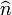$\widehat{n}$ is vector normal to the cylinder surface. The upstream flow is uniform and has no vorticity. The flow is inviscid, incompressible and has constant mass density ρ. The flow therefore remains without vorticity, or is said to be irrotational, with$\nabla \times \vec{V}=0$ everywhere. Being irrotational, there must exist a velocity potential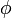$\phi$: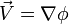$\vec{V}=\nabla\phi$

Being incompressible,$\nabla\cdot\vec{V}=0$, so$\phi$ must satisify Laplace's equation:$\nabla^2\phi=0$

The solution for$\phi$ is obtained most easily in polar coordinates r and θ, related to conventional Cartesian coordinates by x = rcosθ and y = rsinθ. In polar coordinates, Laplace's equation is:$\frac{\partial^2\phi}{\partial r^2} + \frac{1}{r}\frac{\partial \phi}{\partial r} + \frac{1}{r^2} \frac{\partial^2\phi}{\partial \theta^2}=0$

The solution that satisfies the boundary conditions is$\phi(r,\theta)=U\left(r+\frac{R^2}{r}\right)\cos\theta$

The velocity components in polar coordinates are obtained from the components of$\nabla\phi$ in polar coordinates:$V_r=\frac{\partial \phi}{\partial r} = U\left(1-\frac{R^2}{r^2}\right)\cos\theta$

and$V_\theta=\frac{1}{r}\frac{\partial \phi}{\partial \theta} = - U\left(1+\frac{R^2}{r^2}\right)\sin\theta$

Being invisicid and irrotational, Bernoulli's equation allows the solution for pressure field to be obtained directly form the velocity field: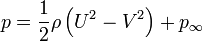$p=\frac{1}{2}\rho\left(U^2-V^2\right) + p_\infty$

where the constants U and$p_\infty$ appear so that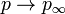$p\rightarrow p_\infty$ far from the cylinder, where V = U. Using$V^2=V_r^2+V_\theta^2$,$p=\frac{1}{2}\rho U^2\left(2\frac{R^2}{r^2}\cos(2\theta)-\frac{R^4}{r^4}\right) + p_\infty$

In the figures, the colorized field referred to as "pressure" is a plot of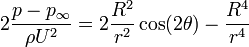$2 \frac{p - p_\infty}{\rho U^2} =2\frac{R^2}{r^2}\cos(2\theta)-\frac{R^4}{r^4}$

On the surface of the cylinder, or r = R, pressure varies from a maximum of 1 (red color) at the stagnation points at θ = 0 and θ = π to a minimum of -3 (purple) on the sides of the cylinder, at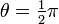$\theta=\tfrac{1}{2}\pi$ and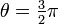$\theta=\tfrac{3}{2}\pi$. Likewise, V varies from V=0 at the stagnation points to V = 2U on the sides, in the low pressure.

###  Stream function

The flow being incompressible, a stream function can be found such that$\vec{V}=\nabla\psi \times \widehat{k}$

It follows from this definition, using vector identities,$\vec{V}\cdot\nabla{\psi}=0$

Therefore a contour of a constant value of ψ will also be a stream line, a line tangent to$\vec{V}$. For the flow past a cylinder, we find:$\psi= U \left( r - \frac{R^2}{r} \right) \sin\theta$

##  Physical interpretation

Laplace's equation is linear, and is one of the most elementary partial differential equations. This simple equation yields the entire solution for both$\vec{V}$ and p because of the constraint of irrotation and incompressibility. Having obtained the solution for$\vec{V}$ and p, the consistency of the pressure gradient with the accelerations can be noted.

The dynamic pressure at the upstream stagnation point has value of ρU2 / 2, a value needed to decelerate the free stream flow of speed U. This same value appears at the downstream stagnation point, this high pressure is again need to decelerate the flow to zero speed. This symmetry arises only because the flow is completely frictionless.

The low pressure on sides on the cylinder is need to provide the centripetal acceleration of the flow.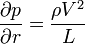$\frac{\partial p}{\partial r}=\frac{\rho V^2}{L}$

where L is the radius of curvature of the flow. But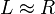$L \approx R$, and$V \approx U$. The integral of the equation for centripetal acceleration, which will over a distance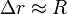$\Delta r \approx R$ will thus yield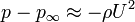$p-p_\infty \approx - \rho U^2$

The exact solution has, for the lowest pressure,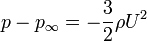$p-p_\infty = - \frac{3}{2} \rho U^2$

The low pressure, which must be present to provide the centripetal acceleration, will also increase the flow speed as the fluid travels from higher to lower values of pressure. Thus we find the maximum speed in the flow, V = 2U, in the low pressure on the sides of the cylinder.

A value of V > U is consistent with conservation of the volume of fluid. With the cylinder blocking some of the flow, V must be greater than U somewhere in the plane through the center of the cylinder and transverse to the flow.

##  Comparison with flow of a real fluid past a cylinder

This symmetry of this ideal solution has the peculiar property of having zero net drag on the cylinder, a property known as D'Alembert's paradox. Unlike an ideal inviscid fluid, a viscous flow past a cylinder, no matter how small the viscosity, will acquire vorticity in a thin boundary layer adjacent to the cylinder. Boundary layer separation can occur, and a trailing wake will occur behind the cylinder. The pressure will be lower on the wake side of the cylinder, than on the upstream side, resulting in a drag force in the downstream direction.